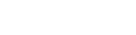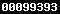### PROSES BERPIKIR SISWA SMA DALAM PENYELESAIAN MASALAH APLIKASI TURUNAN FUNGSI DITINJAU DARI TIPE KEPRIBADIAN TIPOLOGI HIPPOCRATES-GALENUS

#### Sari

Abstract:The aims of the research is to describe the thinking process at senior high school students in solving the application problems of the derivative function viewed from personality type choleric, sanguine, melancholies, and phlegmatic. The research was a descriptive qualitative. The subjects of the research was students of class XII Senior High School 1 Surakarta consist of one student for each personality types. The prosedure of selecting the subjects used purposive sampling. The data of the research was collected by using think aloud method. The validity of the data used was triangulation. Technique analyzing of the data were: (1) classifying the data into three categories: (a) understanding the information, (b) solving the problems, (c) convincing the answer, and then reducing the data, (2) presenting the data, and (3) concluding the data in each categories. The results of the research showed that thinking process for, (1) choleric student: (a) reading and creating an image, understanding information and question, (b) devising solution, connecting the first equation with the planned solution, solving the problem and only using one way solution, (c) substituting the answers into the first equation and determining maximum value of the function, (2) sanguine student: (a) reading and looking, then creating an image, understanding information although having difficulties and understanding question, (b) devising solution, connecting the first equation with the planned solution, solving the problem although having difficulties and using the other solution, (c) substituting the answers into the first equation and adjusting the answers with the problem in question, (3) melancholies student: (a) reading repeatedly, then creating an image, understanding information although having difficulties and understanding question, (b) devising solution, connecting the first equation with the planned solution although having difficulties, solving the problem and using the other solution (c) determining maximum value of the function, (4) phlegmatic student: (a) looking and then creating an image, understanding information and question, (b) devising solution, connecting the first equation with the planned solution although having difficulties, solving the problem and only using one way solution, (c) looking back at the anwers and adjusting with the actual event.

Keywords: thinking process, problem solving, personality type Typology Hippocrates-Galenus

PDF

### Refbacks

• Saat ini tidak ada refbacks.View My Stats